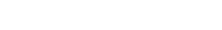# Henri Schurz | Mathematics | SIU

HomeCONTACT

SIU.EDU

## Henri Schurz

### ProfessorP: 618.453.6577
E: hschurz@math.siu.edu
O: Neckers 271
Website

Professor; Ph.D., Humboldt University Berlin, 1997. Stochastic analysis, stochastic dynamical systems, mathematical finance.

## Research Interests

My research focuses on stochastic analysis and its applications. In particular, I am working on the qualitative behavior of stochastic dynamical systems such as systems of stochastic differential equations (ordinary, partial, functional), their numerical methods and stochastic difference equations. The applications range from mechanical engineering (random vibrations, oscillators), laser physics (injection locking), finance (interest rates, dynamic asset pricing), marketing (innovation diffusion) to biology and ecology (random epidemics, population models).

## Selected Publications

1. Applications of numerical methods and its analysis for systems of stochastic differential equations, Bull. Karela Math. Soc. 4 (1) (2007), 1-85.
2. Existence and uniqueness of solutions of semilinear stochastic infinite-dimensional differential systems with H-regular noise, J. Math. Anal. Appl. 332 (1) (2007) 334-345.
3. An axiomatic approach to numerical approximations of stochastic processes, Int. J. Numer. Anal. Model. 3 (4) (2006) 459-480.
4. Stochastic $\alpha$-calculus, a fundamental theorem and Burkholder-Davis-Gundy-type estimates, Dynam. Syst. Applic. 15 (2) (2006) 241-268.
5. Stability of numerical methods for ordinary SDEs along Lyapunov-type and other functions with variable step sizes, Electr. Trans. Numer. Anal. 20 (2005), p. 27-49.
6. Dissipation of mean energy of discretized linear oscillators under random perturbations, Discrete Contin. Dyn. Syst. (Suppl. Vol. 2005), p. 778-783, 2005.
7. Moment attractivity, stability and contractivity exponents of stochastic dynamical systems, Discrete Contin. Dyn. Syst. 7 (3), p. 487-515, 2001.
8. Stability, stationarity, and boundedness of some implicit numerical methods for stochastic differential equations and applications, Logos-Verlag, Berlin, pp. 288, 1997 (Research Monograph, ISBN 3-931216-94-2).